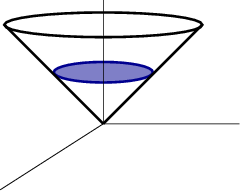# Math Insight

### Image: Plane option for Stokes' theoremFor the curve $\dlc$ formed from intersection of the cone $z^2=x^2+y^2$ and the plane $z=1$, one option is to use the portion of the plane $z=1$ for Stokes' theorem.

Image file: stokes_surface_option_plane.png